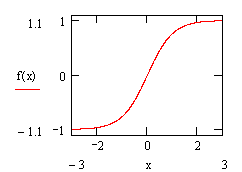﻿ BipolarSigmoidFunction Class# BipolarSigmoidFunction Class

Bipolar sigmoid activation function.Inheritance Hierarchy
SystemObject
Accord.NeuroBipolarSigmoidFunction

Namespace:  Accord.Neuro
Assembly:  Accord.Neuro (in Accord.Neuro.dll) Version: 3.8.0Syntax
```[SerializableAttribute]
public class BipolarSigmoidFunction : IActivationFunction,
ICloneable```

The BipolarSigmoidFunction type exposes the following members.Constructors
NameDescriptionBipolarSigmoidFunction
Initializes a new instance of the SigmoidFunction class.BipolarSigmoidFunction(Double)
Initializes a new instance of the BipolarSigmoidFunction class.
TopProperties
NameDescriptionAlpha
Sigmoid's alpha value.
TopMethods
NameDescriptionClone
Creates a new object that is a copy of the current instance.Derivative
Calculates function derivative.Derivative2
Calculates function derivative.Equals
Determines whether the specified object is equal to the current object.
(Inherited from Object.)Finalize
Allows an object to try to free resources and perform other cleanup operations before it is reclaimed by garbage collection.
(Inherited from Object.)Function
Calculates function value.GetHashCode
Serves as the default hash function.
(Inherited from Object.)GetType
Gets the Type of the current instance.
(Inherited from Object.)MemberwiseClone
Creates a shallow copy of the current Object.
(Inherited from Object.)ToString
Returns a string that represents the current object.
(Inherited from Object.)
TopExtension Methods
NameDescriptionHasMethod
Checks whether an object implements a method with the given name.
(Defined by ExtensionMethods.)IsEqual
Compares two objects for equality, performing an elementwise comparison if the elements are vectors or matrices.
(Defined by Matrix.)To(Type)Overloaded.
Converts an object into another type, irrespective of whether the conversion can be done at compile time or not. This can be used to convert generic types to numeric types during runtime.
(Defined by ExtensionMethods.)ToTOverloaded.
Converts an object into another type, irrespective of whether the conversion can be done at compile time or not. This can be used to convert generic types to numeric types during runtime.
(Defined by ExtensionMethods.)
TopRemarks

The class represents bipolar sigmoid activation function with the next expression:

```               2
f(x) = ------------------ - 1
1 + exp(-alpha * x)

2 * alpha * exp(-alpha * x )
f'(x) = -------------------------------- = alpha * (1 - f(x)^2) / 2
(1 + exp(-alpha * x))^2```

Output range of the function: [-1, 1].

Functions graph:See Also